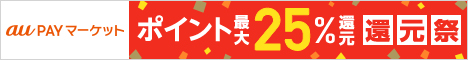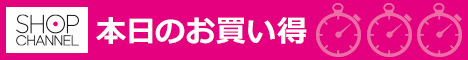# Java 循环结构

## Java 循环结构 – for, while 及 do…while顺序结构的程序语句只能被执行一次。如果您想要同样的操作执行多次,就需要使用循环结构。
Java中有三种主要的循环结构：
● while 循环
● do…while 循环
● for 循环

while 循环

while是最基本的循环，它的结构为：

1 while( 布尔表达式 ) { //循环内容 }

Test.java 文件代码：
1 public class Test { public static void main(String args[]) { int x = 10; while( x < 20 ) { System.out.print(“value of x : “ + x ); x++; System.out.print(“\n“); } } }

1 value of x : 10 value of x : 11 value of x : 12 value of x : 13 value of x : 14 value of x : 15 value of x : 16 value of x : 17 value of x : 18 value of x : 19

do…while 循环

do…while 循环和 while 循环相似，不同的是，do…while 循环至少会执行一次。

1 do { //代码语句 }while(布尔表达式);

Test.java 文件代码：
1 public class Test { public static void main(String args[]){ int x = 10; do{ System.out.print(“value of x : “ + x ); x++; System.out.print(“\n“); }while( x < 20 ); } }

1 value of x : 10 value of x : 11 value of x : 12 value of x : 13 value of x : 14 value of x : 15 value of x : 16 value of x : 17 value of x : 18 value of x : 19

for循环

for循环执行的次数是在执行前就确定的。语法格式如下：

1 for(初始化; 布尔表达式; 更新) { //代码语句 }

●最先执行初始化步骤。可以声明一种类型，但可初始化一个或多个循环控制变量，也可以是空语句。
●然后，检测布尔表达式的值。如果为 true，循环体被执行。如果为false，循环终止，开始执行循环体后面的语句。
●执行一次循环后，更新循环控制变量。
●再次检测布尔表达式。循环执行上面的过程。

Test.java 文件代码：
1 public class Test { public static void main(String args[]) { for(int x = 10; x < 20; x = x+1) { System.out.print(“value of x : “ + x ); System.out.print(“\n“); } } }

1 value of x : 10 value of x : 11 value of x : 12 value of x : 13 value of x : 14 value of x : 15 value of x : 16 value of x : 17 value of x : 18 value of x : 19

Java 增强 for 循环

Java5 引入了一种主要用于数组的增强型 for 循环。
Java 增强 for 循环语法格式如下:

1 for(声明语句 : 表达式) { //代码句子 }

Test.java 文件代码：
1 public class Test { public static void main(String args[]) { int [] numbers = {10, 20, 30, 40, 50}; for(int x : numbers ){ System.out.print( x ); System.out.print(“,”); } System.out.print(“\n“); String [] names ={“James”, “Larry”, “Tom”, “Lacy”}; for( String name : names ) { System.out.print( name ); System.out.print(“,”); } } }

1 10,20,30,40,50, James,Larry,Tom,Lacy,

break 关键字

break 主要用在循环语句或者 switch 语句中，用来跳出整个语句块。
break 跳出最里层的循环，并且继续执行该循环下面的语句。

break 的用法很简单，就是循环结构中的一条语句：

1 break;

Test.java 文件代码：
1 public class Test { public static void main(String args[]) { int [] numbers = {10, 20, 30, 40, 50}; for(int x : numbers ) { // x 等于 30 时跳出循环 if( x == 30 ) { break; } System.out.print( x ); System.out.print(“\n”); } } }

 1 10 20

continue 关键字

continue 适用于任何循环控制结构中。作用是让程序立刻跳转到下一次循环的迭代。

continue 就是循环体中一条简单的语句：

1 continue;

Test.java 文件代码：
1 public class Test { public static void main(String args[]) { int [] numbers = {10, 20, 30, 40, 50}; for(int x : numbers ) { if( x == 30 ) { continue; } System.out.print( x ); System.out.print(“\n“); } } }

 1 10 20 40 50

##### Shell 脚本教程